A New Approach for Torque Ripple Minimization in Brushless DC Motor Using SEPIC Converter | OMICS International
Journal of Electrical & Electronic Systems

# A New Approach for Torque Ripple Minimization in Brushless DC Motor Using SEPIC Converter

S. Sridhar Krishna* and B. Mahesh Kumar

Department of Electrical and Electronics Engineering, Pondicherry Engineering College, Pondicherry, 605014, India

*Corresponding Author:
Sridhar Krishna S
Department of Electrical and Electronics Engineering
Pondicherry Engineering College
Pondicherry, 605014, India
E-mail: sridharkrishna_89[email protected]

Received Date: February 04, 2013; Accepted Date: March 19, 2013; Published Date: March 21, 2013

Citation: Sridhar Krishna S, Mahesh Kumar B (2013) A New Approach for Torque Ripple Minimization in Brushless DC Motor Using SEPIC Converter. J Elec Electron 2:106. doi:10.4172/2167-101X.1000106

Copyright: © 2013 Sridhar Krishna S, et al. This is an open-access article distributed under the terms of the Creative Commons Attribution License, which permits unrestricted use, distribution, and reproduction in any medium, provided the original author and source are credited.

Visit for more related articles at Journal of Electrical & Electronic Systems

#### Abstract

Brushless DC Motors (BLDCM) are widely used in automated industrial applications like Computer Numerical Control (CNC) machinery, aerospace applications and in the field of robotics. But it still suffers from commutation torque ripple, which mainly depends on speed and transient line current in the commutation interval. This work presents a new circuit topology and a DC link voltage control strategy to keep incoming and outgoing phase currents changing at the same rate during commutation. A Single-Ended Primary Inductor Converter (SEPIC) and a switch selection circuit are employed in front of the inverter. The desired commutation voltage is accomplished by the SEPIC converter. The cause of commutation ripple is analyzed, and the way to obtain the desired DC link voltage is introduced in detail. Finally, simulation results show that, compared with the DC-DC converter, the proposed method can obtain the desired voltage much faster and minimize commutation torque ripple more efficiently at both high and low speeds.

#### Keywords

Brushless DC motor (BLDCM); Commutation; DC link voltage control; Hall-Sensor Single-Ended Primary Inductor Converter (SEPIC)

#### Introduction

Brushless DC motor (BLDCM) has been widely used in industrial fields that require high reliability and precise control due to its simple structure, high power density, and extended speeding range [1-3]. The performance of such motors has been significantly improved due to the great development of power electronics, microelectronics, magnetic performance of magnets, and motion control technology in recent years [4-6]. However, commutation torque ripple, which usually occurs due to the loss of exact phase current control, has always been one major factor in preventing BLDCM from achieving high performance. So far, many studies have been performed to reduce commutation torque ripple [7-9]. An original analytical study on commutation torque ripple has been presented in many papers, from which a conclusion has been drawn that relative torque ripple is independent of current and varies with speed. A similar analysis is presented by Niasar , and the strategy of changing the input voltage to reduce commutation torque ripple is proposed.

In Zhang Xi aof eng , a buck converter is used, and commutation torque ripple is then greatly reduced at low speed. In Chen et a l. , a super lift Luo converter is placed at the entrance of the inverter to produce desired DC link voltage, and the structure is more competent under the high-speed work condition, compared with the method proposed by Zhang Xiaofeng . A developed structure of the inverter is proposed by Feipeng et al. , which avoids the effect of the fly-wheeling process and acquires more exact estimated torque with sampling current. In Kumar et al.  Hybrid control has been applied to control BLDC motor whereas in Kuma r  PSO technique has been adopted. All of the above methods suffer from slow voltage adjustment, and therefore, they can only achieve satisfactory torque pulsation suppression in low- or high-speed regions. The proposed method can obtain the desired voltage much faster and minimize commutation torque ripple more efficiently at both high and low speeds.

#### Materials and Methods

Mathematical model of BLDC motor drive system

BLDC motor produces a trapezoidal back-EMF, and therefore the excited current waveform is preferably rectangular-shaped. The phase resistances of the stator windings are assumed to be equal. The self and mutual inductances are constant irrespectively of rotor position due to surface mounted permanent magnet rotor topology. The rotor-induced currents are neglected and the damper windings are also not present. The three phase voltage equation can be expressed as in equation (1):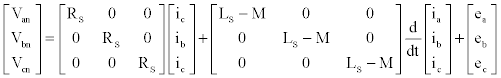(1)

Where

Rs: Phase resistance

Van,Vbn,Vcn: Phase voltages

Ls: Self-inductance

ia, ib, ic: phase currents

M: Mutual inductance

Ea, Eb, Ec: Phase back-EMFs

The equivalent circuit for the BLDC motor is shown in figure 1. Due to the interaction of the currents in stator windings and the magnetic field from rotor magnets, the electromagnetic torque of BLDC motor is produced as follows: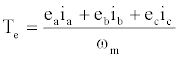(2)

Where ωm is the mechanical speed of the rotor. The equation of motion is given by: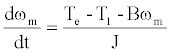(3)

B: damping constant,

J: moment of inertia of rotor shaft and load

For six-step motor control, at each step the instantaneous output power will be will be delivered from two phases connected in series, and is given by:

Po = ωmTe = 2EI (4)

Where I is the current amplitude and E is the induced Back-EMF. From equations (2) and (4), the output torque can also be expressed as:

Te = 2KØI = 2KtI (5)

Analyses of BLDC torque ripples motors due to current commutation

In order to minimize the torque ripples in Brushless DC machines the analysis of torque curves to be performed. The constant current torque waveforms depend on many parameters which are related to design parameters.

The commonly used commutation in 3 phase BLDC motor is the six-step, in which each phase voltage is energized for interval of 120 degree electrical according to the rotor electrical position. At any sector, only one phase is energized as positive and one of the other phases is energized as negative in order to maintain a current path. For control the BLDC motor a typical 3 phase full bridge will be used to drive the motor.

For the analysis of commutation time, the commutations of the current through two phases are to be considered. Phase A will be switched off, and the phase B will replace the A phase and the third phase C will remain conducting.

In this analysis the commutation from phase A to phase B will be considered. The current transfer happens during the six-step, since A phase switch is ON while B phase switch will be OFF, and the third phase switch will remain conducting. In this analysis the transition of conduction from Phase A(+)/C(−) to B(+)/C(−) will be considered as shown in figure 2. In this case the phase A is the de-energized phase and phase B will be the incoming energized phase and phase C is the conducting phase.

Figure 2: Transition of conduction from phase A to B
a. Phase A conducts
b. During commutation time between phase A, B
c. c. Phase B conducts

Ideally the current in BLDC motor with trapezoidal back-EMF is square in shape. Figure 3 shows the ideal current waveforms, yet practically the current in the upcoming phase (ib) takes a finite time to settle to its maximum value, also the die-off phase (ia) takes a finite time to get vanished to zero. This period of commutation between any pair of phases is relatively short comparing to the period of phase conduction.

At each 60 electrical degree, there will be 2 switches Conducting, one from high side of the phase and the other will be from the low side. When phase A and C conducting the high side switch S1 and the low side S4 will be in ON state and current will start to build up. Yet, when switch S1 turned OFF, the current will be decayed through the freewheeling diode and the switch S4, this will take a short time which will occur at each step. In the next sequence where phase B high-side with phase C low- side, the switches S1 and S4 will be in ON state.

Proposed methods for torque ripple minimization

In this work, a SEPIC converter with a switch over MOSFET is used to implement the DC link voltage adjustment, as can be seen in figure 4. In figure 5, S1, S2, and S3 are all power MOSFETs. By operating S1 appropriately, the energy storage components (i.e., L1, L2, C1, and C2) of the SEPIC converter can be adjusted to get the desired output voltage. S2 and S3 are switchover power MOSFETs used for choosing between the input of inverter US and the output voltage of the SEPIC converter UO, which can be calculated as: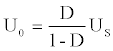(6)

Where D is the duty ratio under the operation of S1.

The three phase voltage equation can be rewritten as:

0 = RiA + LdiA/dt+ eA + UN0

Udc = RiB + LdiB/dt+ eB + UN0

0 = RiC + LdiC/dt+ eC + UN0 (7)

The neutral point voltage can be solved as follows: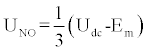(8)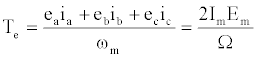(9)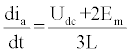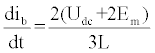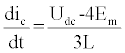(10)

The time taken for ia to vanish from the initial value Im is: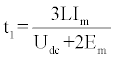(11)

The time taken for ib to increase from 0 to Im is: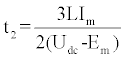(12)

According to (2) (10), and ia + ib + ic = 0, during commutation, the electromagnetic torque can be calculated as: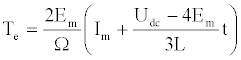(13)

The relative torque ripple is given by: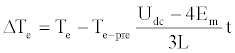(14)

According to (2) and (10) - (13), the following conclusions can be drawn:

1) If Udc>4Em, then t1 > t2, and the torque keeps increasing during commutation.

2) If Udc<4Em, then t1 < t2, and the torque keeps decreasing during commutation.

3) If Udc = 4Em, then t1 = t2, and the torque is constant during commutation.

#### Simulation Results

To verify the results of the proposed strategy in simulations, figure 6 shows the phase current waveform of three phase BLDC motor during transient state without SEPIC converter at an input voltage 200V (DC).

Figure 6: Phase current waveform of three phase BLDC motor without SEPIC during transient period.

Figure 7 shows Torque waveform of three phase BLDC without SEPIC converter at an input voltage 200V (DC), at 0.015 (sec) 50% of load is applied from 0.1(sec) load is decreased from 50% to 30% load.

Figure 7: Torque waveforms of three phase BLDC without SEPIC converter at an input voltage 200V.

Figure 8 shows the DC link output voltage of the BLDC motor, when normal condition input voltage 200 V is given to the motor, during the commutation the output voltage of the SEPIC converter will be applied to motor; hence the torque ripple is greatly reduced.

Figure 9 shows the phase current waveform of three phase BLDC motor during transient state with SEPIC converter at an input voltage 200V (DC).

Figure 9: Phase current waveform of three phase BLDC motor with SEPIC during transient period.

Figure 10 shows Torque waveform of three phase BLDC with SEPIC converter at an input voltage 200V (DC), at 0.015 (sec) 50% of load is applied from 0.1(sec) load is decreased from 50% to 30% load.

Figure 10: Torque waveforms of three phase BLDC with SEPIC converter at an input voltage 200V.

Figure 11 shows Torque and Stator current waveform of three phase BLDC motor without SEPIC converter and its input voltage is 200V, with 30% of load.

Figure 12 shows the improved performance of the SEPIC based BLDC system during 30% load here the torque ripple is reduced from 42% to 8%.

Table 1 shows the Minimized torque ripple in Percentage (%) under various input voltage and speed.

Input Voltage in DC Without SEPIC Torque ripple in % With SEPIC Torque ripple in %
200 V 42 8
250 V 37 6.3
300 V 30 4.3
200 V 47 9
250 V 39 7.2

Table 1: Reduction of Torque Ripple under Various Input Voltage.

BLDC Motor Nameplate data has been shown in appendix I.

#### Conclusion

A circuit topology and control strategy has been proposed to suppress commutation torque ripple of BLDCM in this work. A SEPIC converter is placed at the input of the inverter, and the desired DC link voltage can be achieved by appropriate voltage switch control. No exact value of the commutation interval T is required, and the proposed method can reduce commutation torque ripple effectively within a wide speed range and load. The simulated results show the improved performance of reduction of torque ripple.

#### References

Select your language of interest to view the total content in your interested language

### Article Usage

• Total views: 13457
• [From(publication date):
July-2013 - Jan 25, 2020]
• Breakdown by view type
• HTML page views : 9547

## Review summary

1. kumar
Posted on Oct 11 2016 at 10:10 am
Article is too good and it is useful to me...Can't read the image? click here to refresh